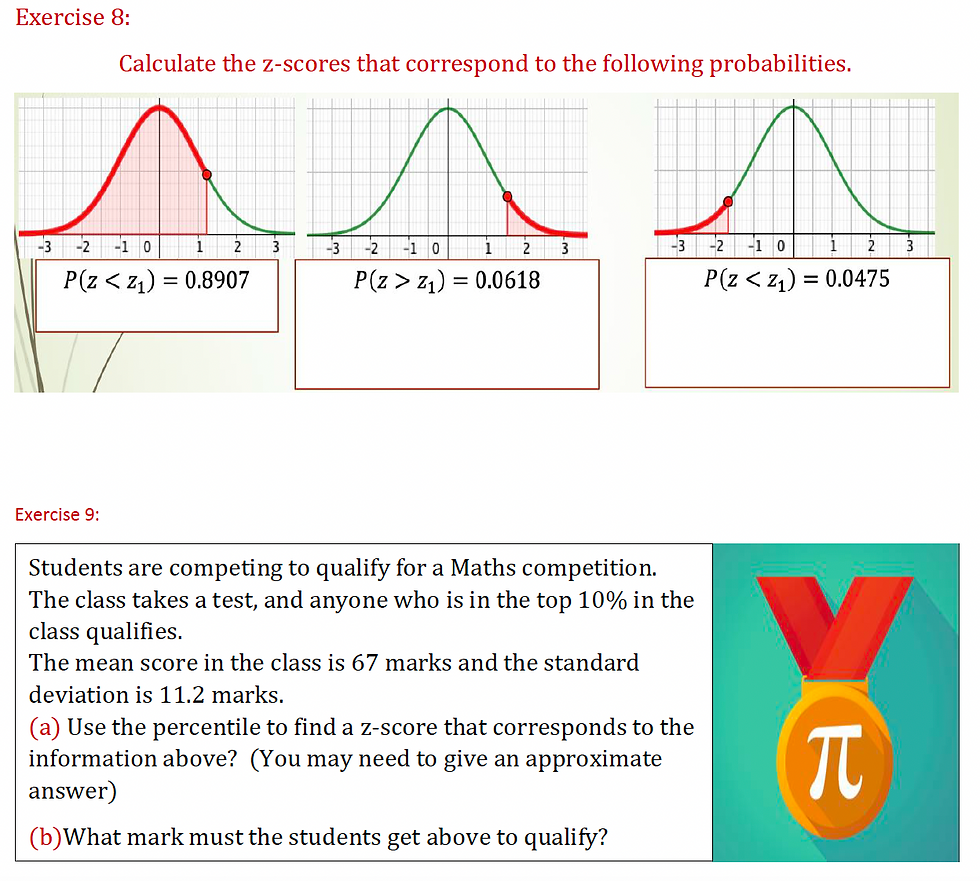top of page
Search

# Inferential Statistics - How to Revise (LCHL)

Inferential Statistics is one of the heavy hitters of the Paper 2 exam.

Every year it comes up as a Part B (50mark) question, and most years a question on Percentiles/Z-scores/P-values etc comes up as a Part A question.

This Blog Post

The Tacit Maths revision worksheet is over 20 pages long.

In this post we will briefly go through the most important sections/exercises of this worksheet.

Also, if you skip to the end, you will see what is (in my opinion) the best LCHL exam question to test your knowledge of Inferential Statistics. There is also a link to the Video Solution of this exam question.

WATERMARK Inferential Statistics
.pdf

Summary (Page 22)This image is useful to give a summary of what's involved in this chapter.

Green Box: I put these in the same box as they are an important part of statistics, but they are not covered in this module.

Red Boxes: This is the key content in the module. All of these individual boxes combine to explain the Central Limit Theorem, and then Hypothesis Testing of a Sample Mean.

Orange Box: I usually highlight that "Proportion Hypothesis Testing" is technically nothing to do with the red boxes.

However, the technique and principles are exactly the same!

Z-Scores/Percentiles (Ex. 2.3 Worksheet: Pg 9)In the worksheet, the build up to explaining z-scores/percentiles might be tricky to follow, so use it however you see fit.

KEY TIP: The exercise above is arguably the most important in the worksheet. My message to students is that there is no point in progressing any further if you cannot complete that exercise.

Z-Scores/Percentiles - Reverse Order (Worksheet: Pg 12-13)This section covers what happens when you are given a percentile, and have to work backwards to find the z-score and the piece of data.

Top Tip: I used often skip this section in previous years. However, if you analyse the exam papers, this technique actually almost every year.

Definitely worth focusing on!

Central Limit Theorem (Worksheet: Pg 14)I've had many sleepless nights trying to find a method for explaining C.L.T.

Even as a teacher, the "concert" examples that I came up with in the worksheet are tricky to explain.

Revelation: It dawned on me that it is actually not hugely important that you understand the theory behind how C.L.T works. It is much more important that they understand the three applications (Confidence Intervals/Test Statistic/P-Values).

I would emphasise this. There's no point stressing yourself out more than you need to be!

The Perfect Exam Question - LC 2019 (Worksheet: Pg 23)

I've analysed all of the LCHL questions, and in my opinion, this one ticks all the boxes.

(a)(i)(ii): Tests your ability to distinguish between Confidence Intervals for a sample PROPORTION V Confidence Intervals for a sample MEAN.

(a)(iii) Test your ability to work backwards from the percentile to the z-score to the data.

(b)(i) Assesses your knowledge on the theory behind P-values

(b)(ii) I always tell students that there will always be questions on the paper that separates the H1/H2 candidates from everyone else. This is that question!